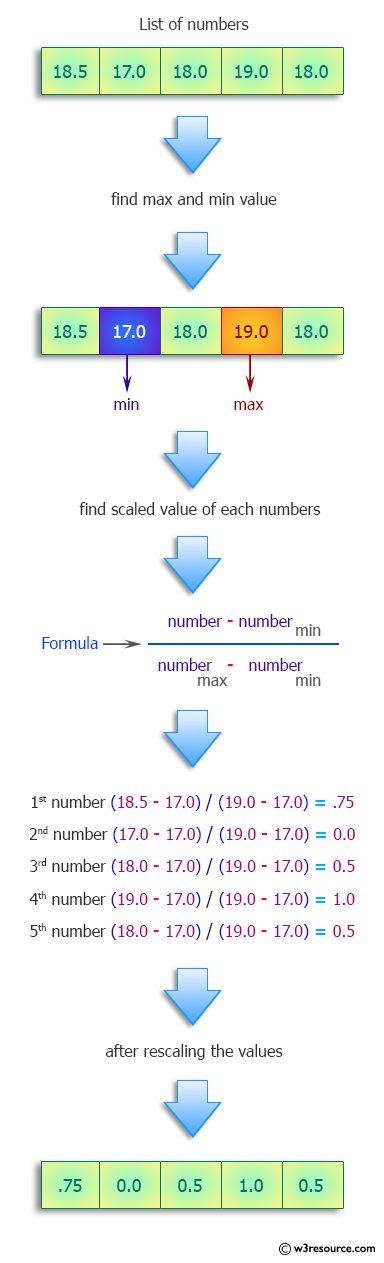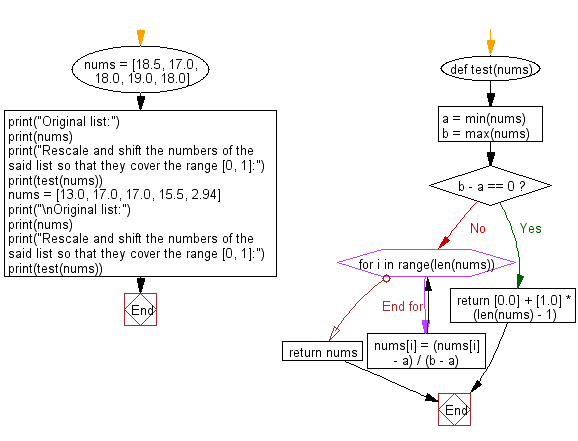﻿ Python: Rescale and shift numbers so that they cover the range [0, 1] - w3resource# Python: Rescale and shift numbers so that they cover the range [0, 1]

## Python Programming Puzzles: Exercise-32 with Solution

Write a Python program to rescale and shift numbers of a given list, so that they cover the range [0, 1].

```Input:
[18.5, 17.0, 18.0, 19.0, 18.0]
Output:
[0.75, 0.0, 0.5, 1.0, 0.5]

Input:
[13.0, 17.0, 17.0, 15.5, 2.94]
Output:
[0.7155049786628734, 1.0, 1.0, 0.8933143669985776, 0.0]
```

Pictorial Presentation:Sample Solution:

Python Code:

``````def test(nums):
a = min(nums)
b = max(nums)
if b - a == 0:
return [0.0] + [1.0] * (len(nums) - 1)
for i in range(len(nums)):
nums[i] = (nums[i] - a) / (b - a)
return nums

nums = [18.5, 17.0, 18.0, 19.0, 18.0]
print("Original list:")
print(nums)
print("Rescale and shift the numbers of the said list so that they cover the range [0, 1]:")
print(test(nums))
nums = [13.0, 17.0, 17.0, 15.5, 2.94]
print("\nOriginal list:")
print(nums)
print("Rescale and shift the numbers of the said list so that they cover the range [0, 1]:")
print(test(nums))
``````

Sample Output:

```Original list:
[18.5, 17.0, 18.0, 19.0, 18.0]
Rescale and shift the numbers of the said list so that they cover the range [0, 1]:
[0.75, 0.0, 0.5, 1.0, 0.5]

Original list:
[13.0, 17.0, 17.0, 15.5, 2.94]
Rescale and shift the numbers of the said list so that they cover the range [0, 1]:
[0.7155049786628734, 1.0, 1.0, 0.8933143669985776, 0.0]
```

Flowchart:## Visualize Python code execution:

The following tool visualize what the computer is doing step-by-step as it executes the said program:

Python Code Editor :

Have another way to solve this solution? Contribute your code (and comments) through Disqus.

What is the difficulty level of this exercise?

Test your Programming skills with w3resource's quiz.

﻿

## Python: Tips of the Day

Clamps num within the inclusive range specified by the boundary values x and y:

Example:

```def tips_clamp_num(num,x,y):
return max(min(num, max(x, y)), min(x, y))
print(tips_clamp_num(2, 4, 6))
print(tips_clamp_num(1, -1, -6))
```

Output:

```4
-1
```